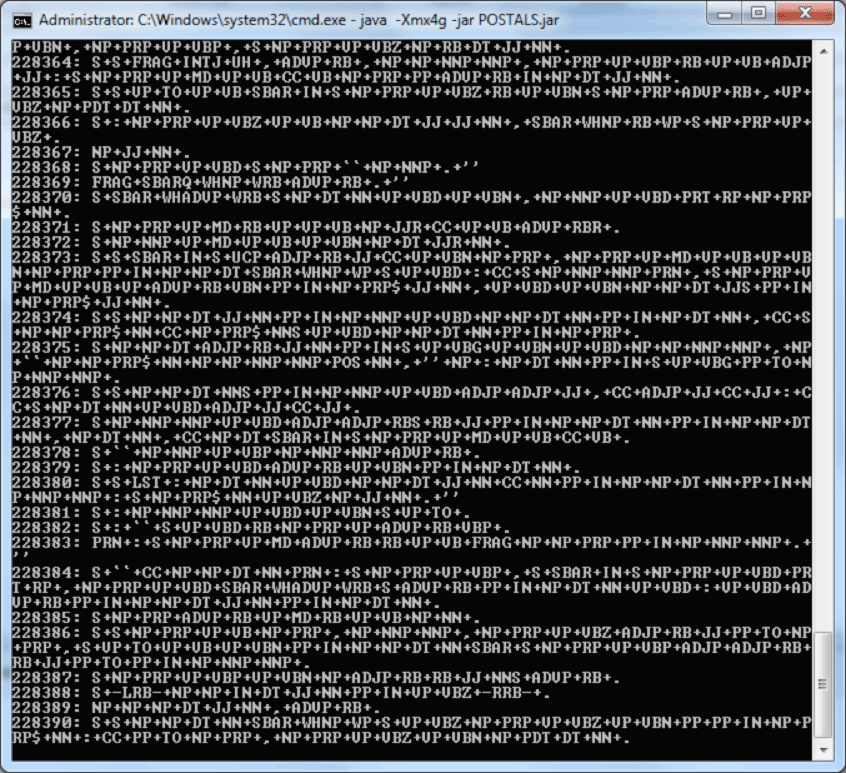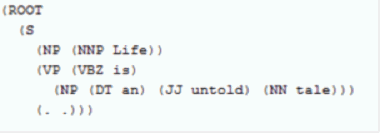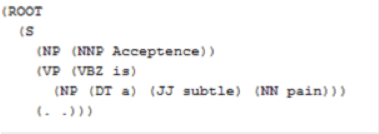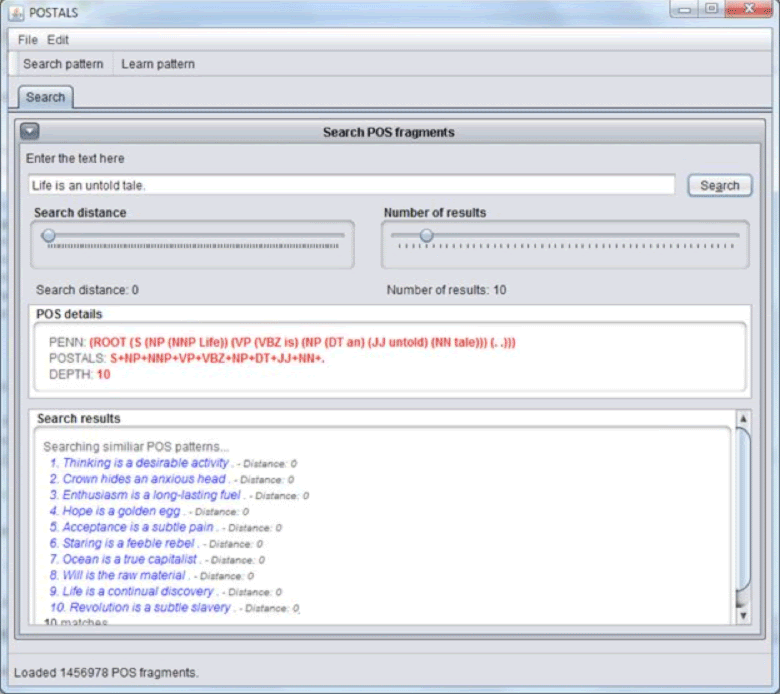## lunes, 23 de septiembre de 2013

### Computational Linguistics and Text Mining

ORIGINAL: DrDobbs
By Frank Jennings
September 19, 2013

Computational Linguistics and Text Mining

A method to fingerprint the structure of English sentences and compute the grammatical distance between fragments.

Sentences in English have patterns that can be identified and extracted through Natural Language Processing (NLP) and computational linguistic techniques. Imagine the immense power a machine would have if it could construct simple or complex sentences by precisely comprehending the technique to construct meaningful sentences. Computational linguistics is a field that has long fascinated me and made me build various NLP-based systems for text extraction and mining. In this article, I explain algorithmically how various text fragments differ from each other.

POS Tagging and Learning
A few months back, I had this interesting idea of prototyping English constructs so that random well-formed sentences can be framed by bots/machines using predefined constructs. One way to achieve this is through computational linguistics and machine learning. I started my journey by analyzing all the "good" sentences in the "world" to build a system that identifies the grammatical structures of these sentences. Every sentence has a grammatical signature, which is not unique, but definitely evident. I've termed this grammatical signature "POSTALS Print." POSTALS (Part of Speech Tagging and Learning System) is an NLP system that I am building that identifies and learns these grammatical signatures. POSTALS Print is just like a fingerprint, with the notable difference that it is not unique.
For instance, consider this sentence:
"Happiness is but an occasional episode in the general drama of pain."

Let us explode this sentence to reveal its structure. I rely on the Penn Treebank bracketing guidelines:
(ROOT (S (NP (NNS Happiness)) (VP (VBZ is) (ADVP (CC but)) (NP (NP (DT an) (JJ occasional) (NN episode)) (PP (IN in) (NP (NP (DT the) (JJ general) (NN drama)) (PP (IN of) (NP (NN pain))))))) (. .)))
Where:

S = Simple declarative cause
NP = Noun Phrase
NNS = Plural Noun
VP = Verb Phrase
VBZ = Third person singular present verb
CC = Coordinating conjunction
NP = Noun Phrase
DT = Determiner
NN = Singular Noun
PP = Prepositional Phrase
IN = Subordinating conjunction As you can see, English sentences can be easily prototyped into a POS tree. Now, let me further flatten the tree to get the POSTALS Print, which is the grammatical signature of the sentence:

(As can be seen, automated identification is not perfect. Here 'happiness' is miscategorized as a plural noun.)

Change any one of the words while maintaining the same word categories and you can create many different sentences that have the same fingerprint.

Web Crawling and Text Mining
To enable the software to construct and validate text fragments, I need to train it with a reasonably accurate and massive text data mined from reliable text corpora on the Web. What is so massive and almost reliable? Wikipedia! If I can successfully extract all possible POSTALS Prints from all the Wikipedia articles, I will have the ability to analyze them and use them as a feed to train the system.

To do this and even extend it farther, I setup a cluster of 8 quad-core dedicated servers to extract and analyze some of the top eBooks from Project Gutenberg, popular Web pages, Wikipedia, and other artifacts from various literature sites. My clustered system, POSTALS, kept analyzing sentences and "learned" the usages of these sentences. (Figure 1)Figure 1: POSTALS in action.
POSTALS also compared different sentences and computed scores similar to the popular Levenshtein distance, which is a string metric for measuring the difference between two sequences.

As I write this article, the system has already understood the construction semantics of text fragments through a set of 27 million sentences extracted from top 100 eBooks from Project Gutenberg and the sources I just mentioned.

A Little Math Now, the biggest challenge for my system is to handle this massive data. Hypothetically, if
N = Count of all possible POS tags,
S Min = A = Count of minimum words in a sentence,
S Max = B = Count of maximum words in a sentence,
Then, the total number of POSTALS Prints T that can be created:
T = NA + NA+1 + NA+2+…NB
So, to evaluate POSTALS Print for sentences having a minimum of 3 words and a maximum of 20 words with a total POS combination of 84 POS tags , we need to allow the system to learn:
843 + 844 + 845+…8420 text fragments.
This is a big number! Thus, POSTALS cannot truly aim at building so many POSTALS Prints. However, the good thing is that not all combinations of POS tags are valid English. In fact, later in my research, I did find the number to be much more manageable.

POS Tags Attract and Repel Each Other! When I allowed POSTALS to crawl the Web, I was primarily focusing on creating POS bigrams for prominent POS tags. I was mostly interested in knowing which POS tags attract each other and which POS tags repel each other. After completing my the crawl through 27-million sentences, I computed a POS bigram distribution without probability computation. This bigram data can be used to computationally generate valid sentences on the go.

For example, from my research data, I know that for having a simple third-person singular present verb (VBZ), I need to assume that the following POS tags appear more frequently before the VBZ tag than do other tags:
[VP=22237435, RB=210375, VBZ=134798, SQ=27674, DT=7853, NN=6526, JJ=2556, CC=2396, SINV=2256, ,=2081, IN=1916, NNP=1527, RBR=1409, NNS=1363, PP=847, PRP=534, VBD=505, RBS=498, CD=402, "=367, JJS=341, NP=233, FW=120, RP=80, JJR=69, NNPS=64, -RRB-=47, VBN=31, TO=27, WRB=18, PRP\$=15, WP=15, ''=12, NX=12, VB=11, WDT=10, :=8, VBP=7, POS=5, UH=1, VBG=1, SYM=0]
And the following POS tags appear more frequently after the VBZ tag:
[NP=8101503, VP=3964786, PP=2115363, ADJP=1994864, ADVP=1851702, S=1422499, SBAR=929467, RB=899301, .=513813, ,=310447, PRT=288317, CC=174890, UCP=44360, PRN=8591, :=4714, WHNP=3703, -RRB-=1920, -LRB-=1268, CONJP=1104, SQ=1059, "=769, ''=615, WHPP=571, NN=551, FRAG=437, SBARQ=257, INTJ=223, VBD=142, NNS=119, JJ=110, NNP=87, WHADJP=72, IN=54, X=23, VBP=13, JJR=9, VBZ=9, QP=8, VB=5, VBN=5, NNPS=2, CD=1, WHADVP=1]

In the above example, VP=22237435 indicates the number occurrences of the tag VP before the tag VBZ is 22 million based on the crawled data.

Just to illustrate this case a little, to construct a valid sentence containing an adjective phrase (ADJP), I use the following bigram distribution to compute the conditional probability of POS tags occurring with each other.
The following POS tags appear more frequently before the ADJP tag:
[VBZ=1721580, DT=1204446, VBD=925102, VB=899263, VBP=833402, NP=726136, RB=711666, CC=485817, NN=362586, ,=343154, S=231026, VBN=199836, PRP=197600, NNS=197390, IN=175953, VBG=155692, JJ=91946, PRP\$=86057, UCP=80301, FRAG=79791, NNP=75007, VP=32206, ADJP=27216, TO=21149, CD=17037, WRB=10900, SINV=8765, JJS=4479, JJR=4040, NNPS=3727, RBR=3627, WP\$=3284, -LRB-=2364, "=2311, :=2304, RRC=2188, RP=1992, START=1542, ADVP=1505, NX=1429, EX=1238, -RRB-=1016, POS=824, FW=715, WHNP=525, SBAR=487, WDT=461, RBS=416, ''=321, SYM=219, PP=140, WP=109, PDT=30, UH=23, MD=14, LS=8, .=1]

And the following POS tags appear more frequently after the ADJP tag:
[JJ=5481218, RB=2569666, ADJP=423890, RBR=380790, RBS=236380, VBN=189200, JJR=147418, ADVP=101392, NP=90829, NN=56058, DT=49134, IN=44174, NNP=44171, JJS=40727, VBG=19958, PRP\$=11235, RP=11029, WHADVP=6822, FW=5976, QP=5477, \$=4369, PRN=4057, CD=3461, VB=3410, VBP=2738, "=1664, CC=1543, PP=1514, VBD=1218, #=1086, ,=1022, WRB=433, NNS=186, -LRB-=60, CONJP=58]

From the above distribution, it is evident that English speakers often use a third-person singular present verbs before an adjective phrase. When I train this system to make it learn a billion POSTALS Prints, it will have the intelligence to deconstruct and construct sentences with precision.

A method to fingerprint the structure of English sentences and compute the grammatical distance between fragments.

Distance Between Text Fragments Having this research as a base, is it possible to find out how two text fragments are different from each other? In information theory, there are known ways to measure the edit distances between two fragments of strings. These techniques are not effective since they don't evaluate the grammatical structure of the text fragments. For instance, Levenshtein proposed a model wherein single-character edits for transforming one text fragment to another is considered as the distance between these fragments). In the method that I devised using computational linguistics techniques, I have found an effective way to find the "grammatical distance" between text fragments.

Consider two similar text fragments, Fragment 1 and Fragment 2:
Grammatical deconstruction of the text based on POSTAL of Fragment 1: Life is an untold tale. generates the results in Figure 2.Figure 2: Life is an untold tale.
Similarly, for Fragment 2: Acceptance is a subtle pain. the result is shown Figure 3.Figure 3: Acceptance is a subtle pain.
Any string-comparison tool or distance-measuring tool will not treat these two fragments as "equal" though they are "grammatically equal." When I say that two text fragments are grammatically equal, they are identical in their POS sequences and ordering as shown in Figures 2 and 3.

Computing the Distance The grammatical distance between these two strings is computed as follows: Both the POSTALS Prints are overlaid and the distance of one POS tag to the corresponding node in the other fragment is computed considering lesser weighting of distance for the earlier POS tags to a greater weighting of distance for the later POS tags.
Here is a reference implementation in Java:?

private static int getPostalsDistance(String s1, String s2) {
String pennString1 = getPennString(s1);
String cleanPenn1 = getCleanPenn(pennString1);
String pennString2 = getPennString(s2);
String cleanPenn2 = getCleanPenn(pennString2);
StringTokenizer stok1 = new StringTokenizer(cleanPenn1, "+");
ArrayList array1 = new ArrayList();
ArrayList array2 = new ArrayList();
while (stok1.hasMoreTokens()) {
}

StringTokenizer stok2 = new StringTokenizer(cleanPenn2, "+");
while (stok2.hasMoreTokens()) {
}

int postalsDistance = 0;
//Find the shorter pattern
if (array1.size() < array2.size()) {
for (int i = 0; i < array1.size(); i++) {
String p1 = (String) array1.get(i);
String p2 = (String) array2.get(i);
if (!p1.equals(p2)) {
postalsDistance += i;
}
}

} else {

for (int i = 0; i < array2.size(); i++) {
String p1 = (String) array2.get(i);
String p2 = (String) array1.get(i);
if (!p1.equals(p2)) {
postalsDistance += i;
}
}
}
return postalsDistance;
What is more interesting is that when I have the ability to compute the POSTALS distance between two random text fragments, I can enable the system to construct similar sentences based on the POSTALS distance. I have built a GUI which, based on the input sentence and the POSTALS distance, generates random sentences (Figure 4).Figure 4: GUI for the POSTALS system.
Conclusion Using a combination of computational linguistics and text mining, we can build a complex text-generating system that can "talk" and "respond" like humans when trained effectively. What is the need of computing the POSTALS Print? How will this information be useful in linguistic studies? I believe:

In future, machines will have the ability to construct simple-to-complex English sentences with almost 100% accuracy provided the mined text base is reasonably huge and accurate.
The machines will start "understanding" the mood of sentences and be able to group sentences based on mood and other factors.
A grammar-checking system that flags bad grammar and auto-suggests right structures purely based on mined data is possible.
An AI-based learning system that can "understand" and "communicate" with us in English is possible. When they do this, this kind of fingerprinting and categorization of sentences will play a crucial part in their linguistic capabilities.

Frank Jennings works as a software engineer for Adobe.

#### Publicar un comentario

Nota: solo los miembros de este blog pueden publicar comentarios.